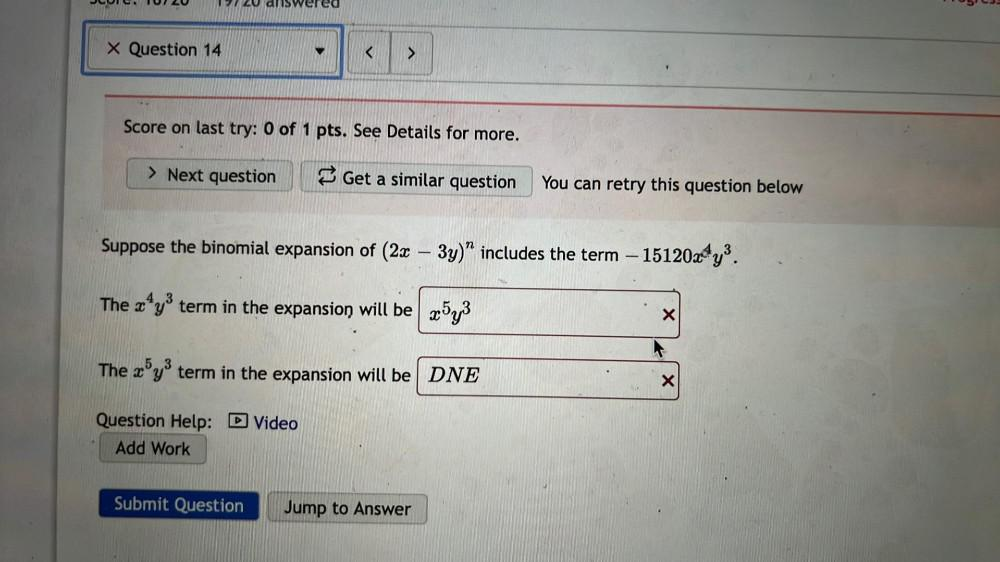Question:

# Suppose the binomial expansion of (2x – 3y)^n includes the term –15120x^4y^3 The x^4y^3 term in the expansion will be ___ The x^5y^3 term in the expansion will be ___Suppose the binomial expansion of (2x – 3y)^n includes the term –15120x^4y^3 The x^4y^3 term in the expansion will be ___ The x^5y^3 term in the expansion will be ___